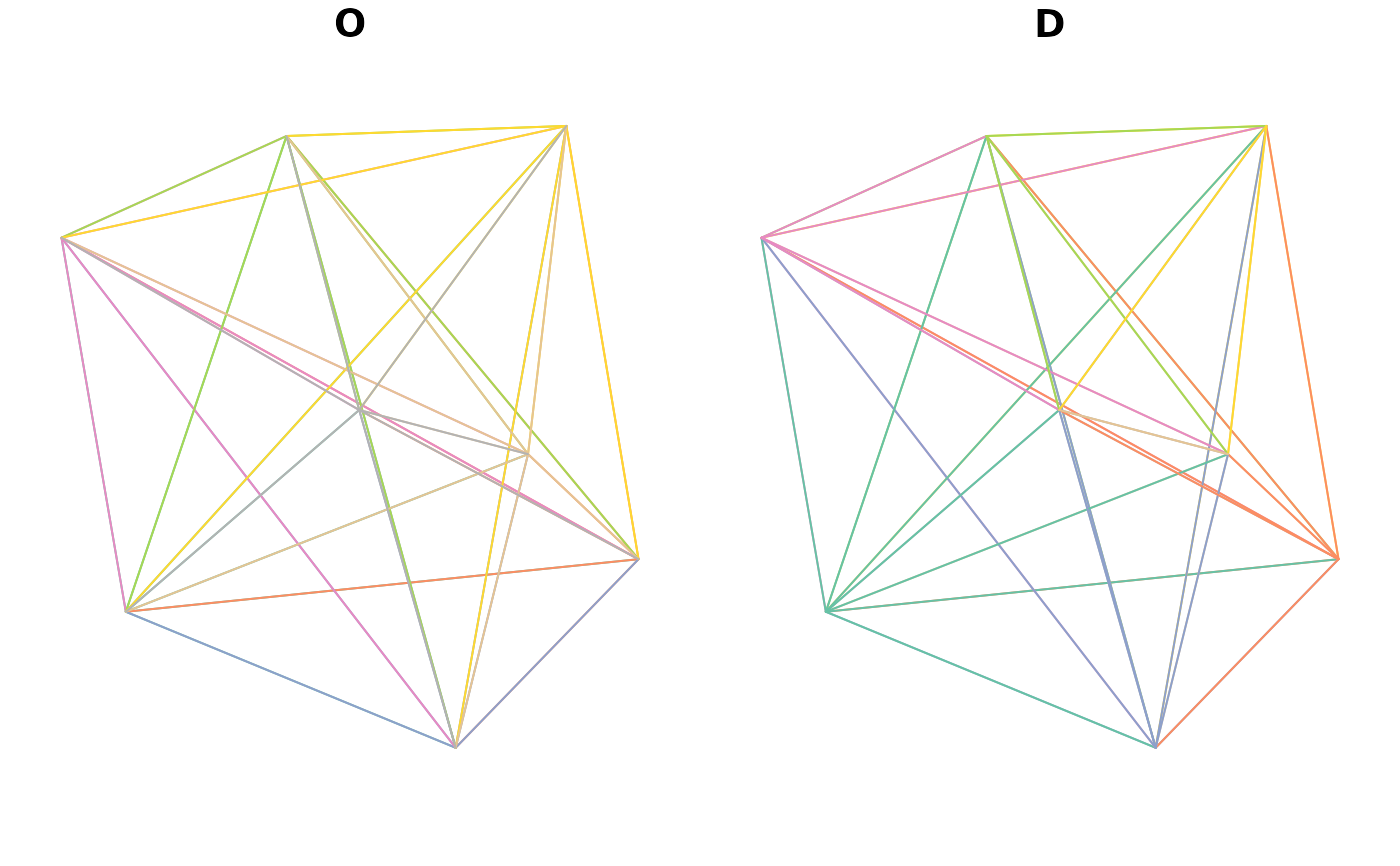This function makes that makes the creation of `sf` objects with LINESTRING geometries easy.

## Usage

``points2line(p)``

## Arguments

p

A spatial (points) obect or matrix representing the coordinates of points.

Other lines: `angle_diff()`, `geo_toptail()`, `is_linepoint()`, `line2df()`, `line2points()`, `line_bearing()`, `line_breakup()`, `line_midpoint()`, `line_segment()`, `line_via()`, `mats2line()`, `n_vertices()`, `onewaygeo()`, `toptail_buff()`
``````l_sf <- points2line(cents_sf)